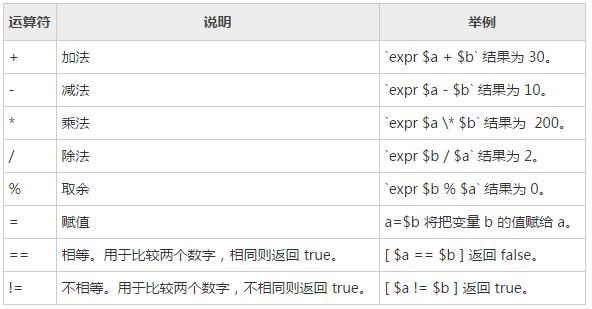算数运算符

#!/bin/sh
a=10
b=20
val=`expr \$a + \$b`
echo "a + b : \$val"
val=`expr \$a - \$b`
echo "a - b : \$val"
val=`expr \$a \* \$b`
echo "a * b : \$val"
val=`expr \$b / \$a`
echo "b / a : \$val"
val=`expr \$b % \$a`
echo "b % a : \$val"
if [ \$a == \$b ]
then
echo "a is equal to b"
fi
if [ \$a != \$b ]
then
echo "a is not equal to b"
fi

a + b : 30
a - b : -10
a * b : 200
b / a : 2
b % a : 0
a is not equal to b

• 乘号前边必须加反斜杠()才能实现乘法运算；
• if...then...fi 是条件语句，后续将会讲解。
• 条件表达式要放在方括号之间，并且要有空格，例如 [\$a==\$b] 是错误的，必须写成 [ \$a == \$b ]